### 一、使用Numpy实现机器学习

1. 首先，给出一个数组x，然后基于表达式y=3x2+2，加上一些噪音数据到达另一组数据y。
2. 然后，构建一个机器学习模型，学习表达式y=wx2+b的两个参数w、b。利用数组x，y的数据为训练数据。
3. 最后，采用梯度梯度下降法，通过多次迭代，学习到w、b的值。

1）导入需要的库

import numpy as np
from matplotlib import pyplot as plt
%matplotlib inline

2）生成输入数据x及目标数据y

np.random.seed(666)
x = np.linspace(-1, 1, 100).reshape(100, 1)
y = 3 * np.power(x, 2) + 2 + 0.2 * np.random.rand(x.size).reshape(100, 1)

3）查看x、y数据分布情况

# 绘图
plt.scatter(x,y)
plt.show()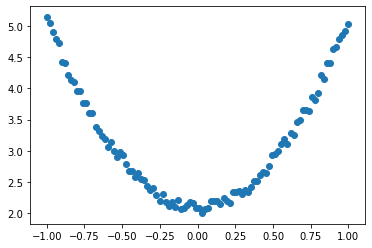4）初始化权重参数

# 随机初始化参数
w = np.random.rand(1,1)
b = np.random.rand(1,1)

5）训练模型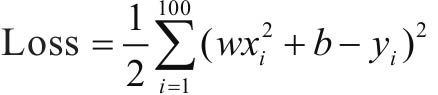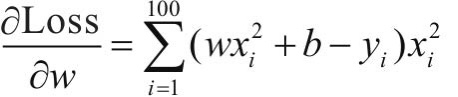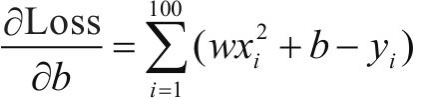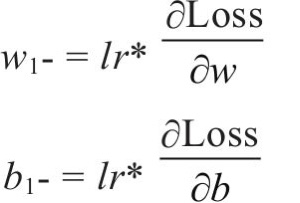lr = 0.001 # 学习率
for i in range(800):
# 前向传播
y_pred = np.power(x, 2) * w + b
# 定义损失函数
loss = 0.5 * (y_pred - y) ** 2
loss = loss.sum()
# 计算梯度
grad_w = np.sum((y_pred - y) * np.power(x, 2))
# 使用梯度下降法，是loss最小
b -= lr * grad_b

6）可视化结果

plt.plot(x, y_pred, 'r-', label='predict')
plt.scatter(x, y, color='blue', marker='o', label='true')

plt.xlim(-1, 1)
plt.ylim(2, 6)
plt.legend()
plt.show()
print(w, b)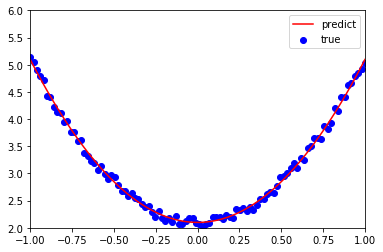[[2.99850472]] [[2.0989827]]

1）导入需要的库

import torch as t
from matplotlib import pyplot as plt
%matplotlib inline

2）生成训练数据，并可视化数据分布情况

# 随机种子
torch.manual_seed(666)

# 生成x坐标数据，x为tensor，需要把x的形状装换为100×1
x = torch.unsqueeze(torch.linspace(-1, 1, 100), dim=1)
# 生成y坐标数据，y为tensor，形状为100×1，另加上一些噪声
y = 3 * x.pow(2) + 2 + 0.2 * torch.rand(x.size())

# 绘图，把tensor数据转换为numpy数据
plt.scatter(x.numpy(), y.numpy())
plt.show()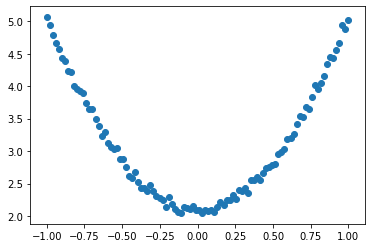3）初始化权重参数

# 随机初始化参数，参数w，b为需要学习的，故需requires_grad=True
w = torch.randn(1, 1, dtype = torch.float, requires_grad=True)
b = torch.zeros(1, 1, dtype = torch.float, requires_grad=True)

4）训练模型

lr = 0.001 # 学习率
for i in range(800):
# 前向传播
y_pred = x.pow(2).mm(w) + b
# 定义损失函数
loss = 0.5 * (y_pred - y) ** 2
loss = loss.sum()
loss.backward()

# 梯度清零
b.grad.zero_()

5）可视化训练结果

plt.plot(x.numpy(), y_pred.detach().numpy(), 'r-', label='predict') # predict
plt.scatter(x.numpy(), y.numpy(), color='blue', marker='o', label='true') # true data

plt.xlim(-1, 1)
plt.ylim(2, 6)
plt.legend()
plt.show()
print(w, b)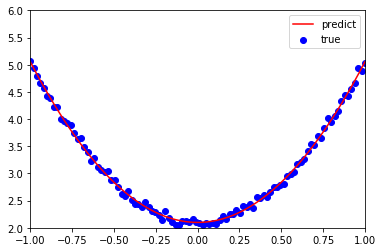tensor([[2.9578]], requires_grad=True) tensor([[2.0990]], requires_grad=True)

12-291万+

#### Pytorch之Tensor和Numpy之间的转换

07-313万+

#### Pytorch学习笔记（二）使用Pytorch的常见错误汇总08-083141

#### 深入Pytorch中的Tensor，梯度以及权重

05-098788

10-211108

01-2657

#### 【pytorch】pytorch学习笔记（一）

06-126629

#### 一文读懂PyTorch张量基础（附代码）

09-17329

#### pytorch与tensorflow的numpy与tensor互转

04-194277

02-27482©️2020 CSDN 皮肤主题: 终极编程指南 设计师: CSDN官方博客点击重新获取扫码支付1.余额是钱包充值的虚拟货币，按照1:1的比例进行支付金额的抵扣。
2.余额无法直接购买下载，可以购买VIP、C币套餐、付费专栏及课程。余额充值